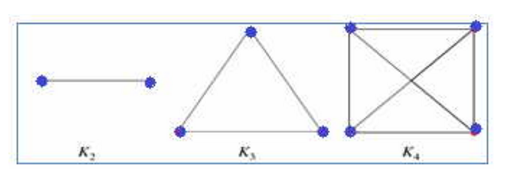# Edges present in Complete Graph with N Vertices - Data Structure

Q.  How many edges are present in a Complete Graph with ' N ' Vertices?
- Published on 27 Aug 15

a. N - 1
b. N - 1 / 2
c. N ( N - 1 ) / 2
d. ( N - 1 )2

ANSWER: N ( N - 1 ) / 2

#### Discussion

• Nirja Shah   -Posted on 18 Nov 15
The complete graph with n vertices is denoted by K n and has N ( N - 1 ) / 2 undirected edges. In complete graph every pair of distinct vertices is connected by a unique edge.
Example.Suppose that in a graph there is 25 vertices, then the number of edges will be 25(25 -1)/2 = 25(24)/2 = 300

## ➨ Post your comment / Share knowledgeEnter the code shown above:

(Note: If you cannot read the numbers in the above image, reload the page to generate a new one.)